Sie sind hier: ICP » R. Hilfer » Publikationen

# III Dielectric Response

[63.2.1.1] The low-frequency dielectric response of a porous medium is influenced by the randomness in its pore-space geometry. [63.2.1.2] Given the geometric description of the pore space developed in the last section, two questions arise: [63.2.1.3] One is how the geometrical information encapsuled in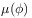and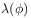expresses itself in the effective complex dielectric constant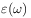, where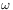is the frequency. [63.2.1.4] This will be walled the direct problem. [63.2.1.5] The inverse problem is the question what geometric information can be obtained from a measurement of the frequency-dependent dielectric constant.

[63.2.2.1] To study these two questions, standard effective-medium theory is an appropraite tool for a first approximate investigation. [63.2.2.2] Consider again the subdivision of the porous medium into cells of length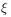. [63.2.2.3] Let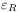and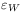be the complex frequency-dependent dielectric constants of the constituent materials where the index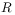stands for the rock matrix and the index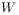stands for water (or brine) filling the pore space. [63.2.2.4] Because alle cells are statistically independent, standard one-cell effective-medium theory can be employed to write a self-consistency equation for the effective dielectric consantof the medium, which reads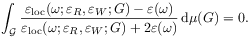(3.1)

[page 64, §0]    [64.1.0.1] Here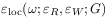is the local effective dielectric constant, which depends on the constituent materialsandand the local geometry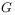. [64.1.0.2] The integration is performed over the space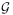of all possible local geometries, and the probability measure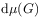onrepresents a complete description of the statistical pore-space geometry.

[64.1.1.1] It was discussed in Section II that a complete geometric description such asis not known, and that in the present paper the local porosity distributionand the local percolation probabilitywill be used as approximate descriptions. [64.1.1.2] This implies that the local effective dielectric constantmust be replaced simultaneously by an approximate effective local dielectric constant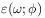. [64.1.1.3] It depends on the local porosity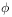as the only geometrical quantity. [64.1.1.4] As a consequence of the basic hypothesis of local simplicity discussed above,can later be approximated by simple geometric models of the pore space. [64.1.1.5] Note that the dependence onandas well as the index “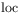” have been suppressed to shorten the notation. [64.1.1.6] With these simplifications one arrives at the simpler equations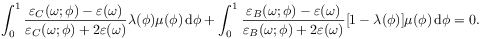(3.2)

[64.1.1.7] Here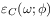and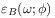are the local effective dielectric constants. [64.1.1.8] The index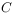stands for conducting (percolating) local geometries and the index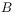for blocking (nonpercolating) cells.

[64.1.2.1] Equation (3.2) represents the starting point for the present investigation, and it is appropriate to discuss briefly its content. [64.1.2.2] Mathematically, eq. (3.2) is a complicated integral equation involving five different functions. [64.1.2.3] The average local dielectric properties,are assumed to be known. [64.1.2.4] According to the hypothesis of local simplicity, their precise form is not sensitive to geometric properties other than porosity, and thus they will in practice be determined from very simple geometrical models of the local pore space. [64.1.2.5] The important assumptions implicit in using equation (3.2) are twofold: [64.1.2.6] The first is that local geometries are uncorrelated. [64.1.2.7] The second says that the important geometric features giving rise to dielectric dispersion can be described using local porosity distributions and local percolation probabilities. [64.1.2.8] With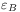and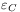known, eq. (3.2) can be written as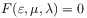. [64.1.2.9] The direct problem is to determine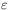given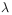and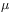as geometrical input. [64.1.2.10] The inverse problems are to calculatefromandor to findgivenand.

[64.1.3.1] Before embarking on the discussion of these problems, some notation and conventions must be established for the subsequent treatment.